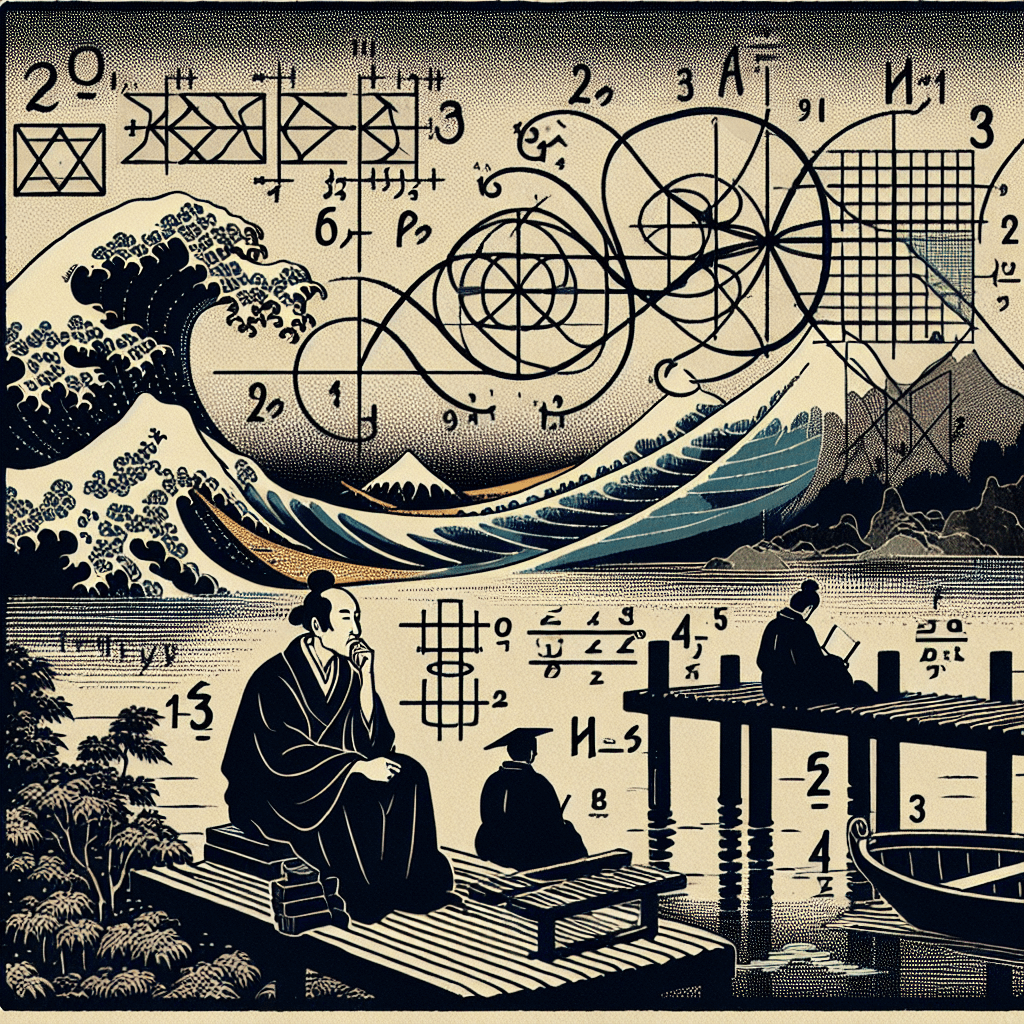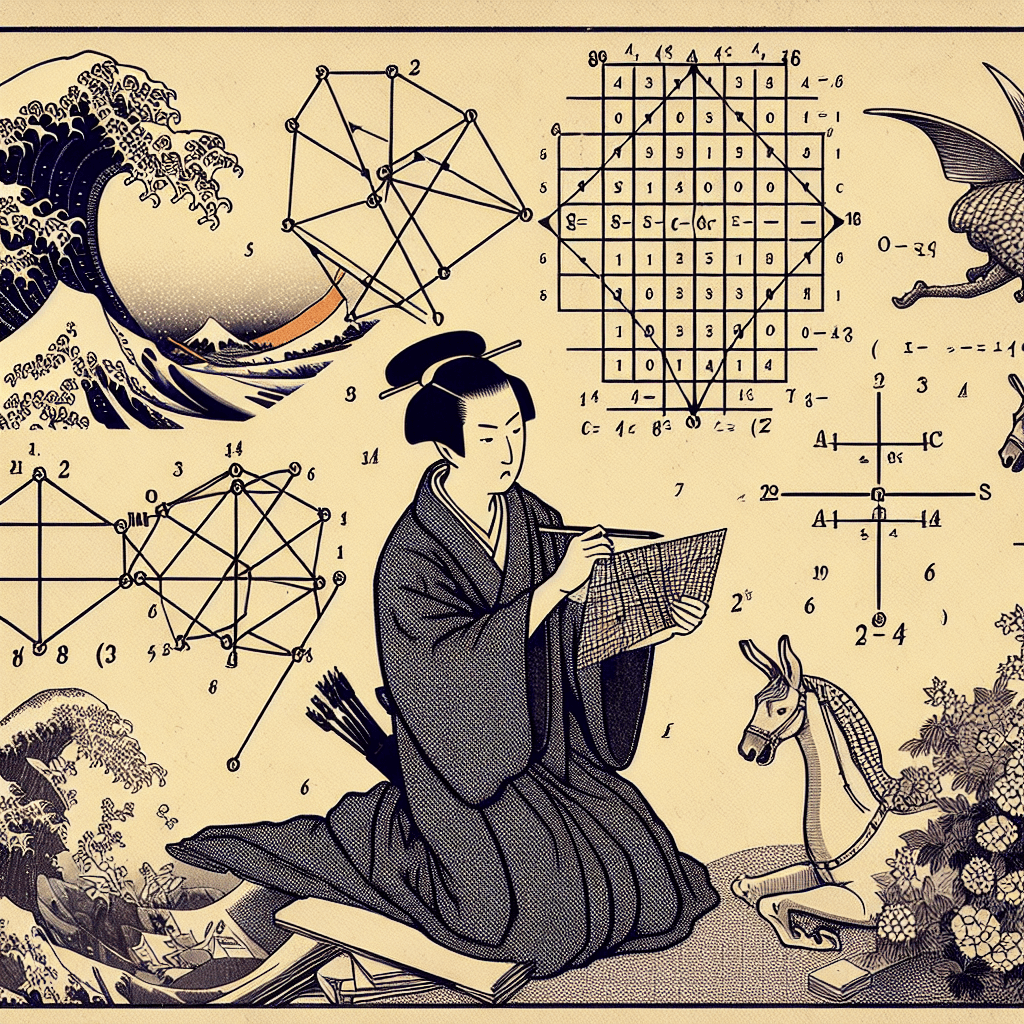# Primes, the Zeta Function, and the Riemann Hypothesis: An Insightful Journey

syndu | Nov. 3, 2023, 10:06 a.m.## Primes, the Zeta Function, and the Riemann Hypothesis: An Insightful Journey

The world of mathematics is a fascinating one, filled with intricate patterns, complex theories, and profound insights. Among these, prime numbers and the Riemann Hypothesis about the zeta function hold a special place. They are fundamental to our understanding of number theory and have implications that stretch far beyond the realm of pure mathematics.

### Prime Numbers

Prime numbers are the building blocks of the number system. A prime number is a natural number greater than 1 that has no positive divisors other than 1 and itself. The first few prime numbers are 2, 3, 5, 7, 11, and so on. They are the atoms of arithmetic - every natural number can be uniquely factored into primes.

Prime numbers have fascinated mathematicians for centuries due to their seemingly random distribution. Despite their randomness, they exhibit a certain pattern, or rather, a lack of pattern, which is the subject of the Prime Number Theorem. This theorem states that the probability that a randomly chosen number n is prime is roughly 1 / log(n), where log is the natural logarithm.

### The Zeta Function

The Riemann Zeta function, named after Bernhard Riemann, is a complex function that encodes information about prime numbers in a surprisingly intricate way. It is defined for complex numbers s with real part greater than 0, and it extends to a meromorphic function on the whole complex plane, except for a simple pole at s=1. The function is defined as:

``````
ζ(s) = 1^(-s) + 2^(-s) + 3^(-s) + 4^(-s) + ...
``````

The Zeta function has a deep connection with prime numbers. The Euler Product Formula shows this relationship by expressing the Zeta function as an infinite product over all primes:

``````
ζ(s) = (1/1-2^(-s)) * (1/1-3^(-s)) * (1/1-5^(-s)) * ...
``````

### The Riemann Hypothesis

The Riemann Hypothesis, proposed by Bernhard Riemann in 1859, is one of the most important unsolved problems in mathematics. It is connected with the distribution of prime numbers and is centered around the zeros of the Zeta function.

Riemann discovered that the Zeta function has trivial zeros at all negative even integers. However, it also has non-trivial zeros in the critical strip where the real part of s is between 0 and 1. The Riemann Hypothesis asserts that all non-trivial zeros of the Zeta function lie on the critical line where the real part of s is 1/2.

This hypothesis, if proven true, would have profound implications for number theory, particularly in understanding the distribution of prime numbers. Despite many efforts, it remains unproven and is one of the seven "Millennium Prize Problems" for which the Clay Mathematics Institute offers a \$1 million reward for a correct solution.

### Conclusion

Prime numbers and the Riemann Hypothesis about the Zeta function are two of the most captivating topics in mathematics. They embody the beauty, complexity, and mystery inherent in the mathematical world. While we have made significant strides in understanding these concepts, there is still much to be discovered, and the journey of exploration continues.

##### Lilith's Grimoire

The app you are using right now is Lilith's Grimoire

The Grimoire is full of Source Code. It is alive, and running, and you can explore the way it is built

The first lesson uncovers all of it.#### The Prime Number Theorem and its Implications for Cryptography and the Crypto Economy#### The Delicate Balance: Self-Defense and Non-Violence in Various Traditions and Religions#### The Nagual: A Journey into the Unseen#### The Power of Silence: A Journey into the Unknown#### Understanding and Calculating Determinants#### The Art of Understanding Understanding: A Reflection on Conversations with Lilith#### Basics of Linear Algebra: Vectors, Matrices, and Operations#### The Future of Personalized Medicine: A Boost from Artificial Intelligence